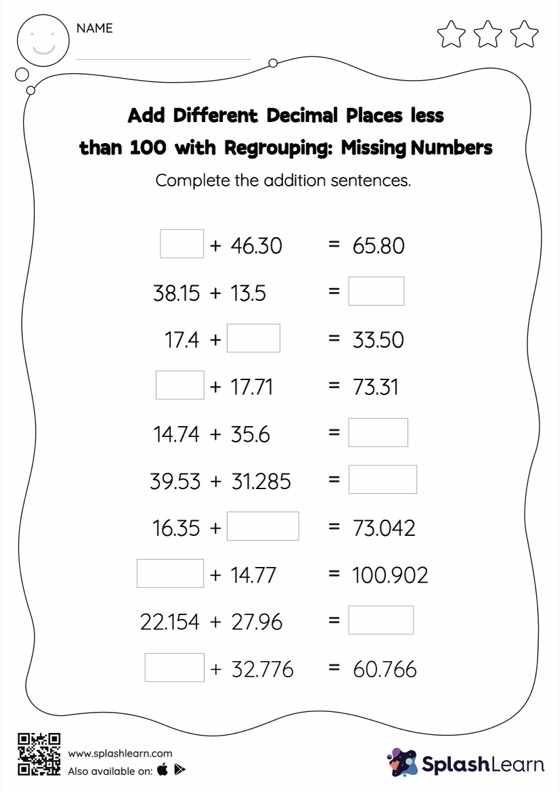# Add Different Decimal Places less than 100 with Regrouping: Missing Numbers Worksheet

Home > Add Different Decimal Places less than 100 with Regrouping: Missing NumbersCan your young mathematician add different decimal places less than 100 with regrouping? That's exactly what they do here. Students use the relationship between addition and subtraction to find the missing number while adding decimals. To reach the answer, they must also regroup the numbers in add different decimal places less than 100 with regrouping worksheet using the relationships between ones, tenths, hundredths, thousandths, etc.